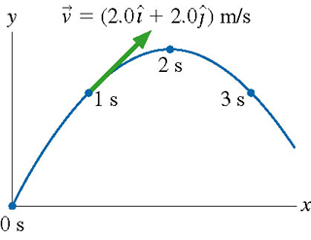# Problem: A physics student on Planet Exidor throws a ball, and it follows the parabolic trajectory shown in the figure . The balls position is shown at 1s intervals until exttip{t}{t} =3s. At exttip{t}{t} =1s, the balls velocity is exttip{vec{v}}{v_vec} =( 2.0+ 2.0 )/s.Determine the balls velocity at exttip{t}{t} =0.Determine the balls velocity at exttip{t}{t} =2s.Determine the balls velocity at exttip{t}{t} =3s.What is the value of exttip{g}{g} on Planet Exidor?What was the balls launch angle?

###### FREE Expert Solution
95% (322 ratings)
###### Problem Details

A physics student on Planet Exidor throws a ball, and it follows the parabolic trajectory shown in the figure. The balls position is shown at 1s intervals until =3s. At =1s, the balls velocity is =( 2.0+ 2.0 )/s.

Determine the balls velocity at =0.

Determine the balls velocity at =2s.

Determine the balls velocity at =3s.

What is the value of on Planet Exidor?

What was the balls launch angle?

Frequently Asked Questions

What scientific concept do you need to know in order to solve this problem?

Our tutors have indicated that to solve this problem you will need to apply the Projectile Motion: Positive Launch concept. If you need more Projectile Motion: Positive Launch practice, you can also practice Projectile Motion: Positive Launch practice problems.